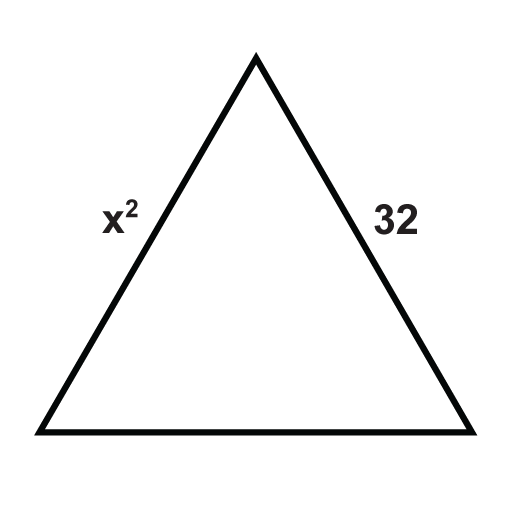# 4.5: Equilateral Triangles

•• Contributed by CK12
• CK12

Properties of triangles with three equal sides.

Equilateral Triangle Theorem: All equilateral triangles are also equiangular. Furthermore, all equiangular triangles are also equilateral.Figure $$\PageIndex{1}$$

If $$\overline{AB}\cong \overline{BC}\cong \overline{AC}$$, then $$\angle A\cong \angle B\cong \angle C$$. Conversely, if $$\angle A\cong \angle B\cong \angle C$$, then $$\overline{AB}\cong \overline{BC}\cong \overline{AC}$$.

What if you were presented with an equilateral triangle and told that its sides measure $$x$$, $$y$$, and 8? What could you conclude about $$x$$ and $$y$$?

Example $$\PageIndex{1}$$

Fill in the proof:

Given: Equilateral $$\Delta RST$$ with

$$\overline{RT}\cong \overline{ST}\cong \overline{RS}$$

Prove: $$\Delta RST$$ is equiangularFigure $$\PageIndex{2}$$

Solution

Statement Reason
1. 1. Given
2. 2. Base Angles Theorem
3. 3. Base Angles Theorem
4. 4. Transitive $$PoC$$
5. $$\Delta RST$$ is equiangular 5.
Statement Reason
1. $$RT\overline{AB}\cong ST\overline{AB}\cong RS\overline{AB}$$ 1. Given
2. $$\angle R\cong \angle S$$ 2. Base Angles Theorem
3.$$\angle T\cong \angle R$$ 3. Base Angles Theorem
4. $$\angle T\cong \angle S$$ 4. Transitive $$PoC$$
5. $$\Delta RST$$ is equiangular 5. Definition of equiangular.

Example $$\PageIndex{2}$$

True or false: All equilateral triangles are isosceles triangles.

Solution

This statement is true. The definition of an isosceles triangle is a triangle with at least two congruent sides. Since all equilateral triangles have three congruent sides, they fit the definition of an isosceles triangle.

Example $$\PageIndex{3}$$

Find the value of $$x$$.Figure $$\PageIndex{3}$$

Solution

Because this is an equilateral triangle $$3x−1=11$$. Solve for $$x$$.

\bgin{align*} 3x−1&=11 \\3x&=12 \\ x&=4 \end{align*}

Example $$\PageIndex{4}$$

Find the values of $$x$$ and $$y$$.Figure $$\PageIndex{3}$$

Solution

The markings show that this is an equilateral triangle since all sides are congruent. This means all sides must equal $$10$$. We have $$x=10$$ and $$y+3=10$$ which means that $$y=7$$.

Example $$\PageIndex{5}$$

Two sides of an equilateral triangle are $$2x+5$$ units and $$x+13$$ units. How long is each side of this triangle?

Solution

The two given sides must be equal because this is an equilateral triangle. Write and solve the equation for $$x$$.

\egin{align*}2x+5 &=x+13 \\ x&=8 \end{align*}

To figure out how long each side is, plug in 8 for $$x$$ in either of the original expressions. $$2(8)+5=21$$. Each side is $$21$$ units.

### Review

The following triangles are equilateral triangles. Solve for the unknown variables.

1.Figure $$\PageIndex{4}$$
2.Figure $$\PageIndex{5}$$
3.Figure $$\PageIndex{6}$$
4.Figure $$\PageIndex{7}$$
5.Figure $$\PageIndex{8}$$
6.Figure $$\PageIndex{9}$$
7.Figure $$\PageIndex{10}$$
8.Figure $$\PageIndex{11}$$
9.Figure $$\PageIndex{12}$$
10.Figure $$\PageIndex{13}$$
11.Figure $$\PageIndex{14}$$
12.Figure $$\PageIndex{15}$$
13.Figure $$\PageIndex{16}$$
14.Figure $$\PageIndex{17}$$
15. Find the measures of $$x$$ and $$y$$.Figure $$\PageIndex{18}$$

Interactive Element

Video: Equilateral Triangles Principles - Basic

Activities: Equilateral Triangles Discussion Questions

Study Aids: Equilateral Triangles Discussion Questions

Practice: Equilateral Triangles

Real World: Equilateral Triangles